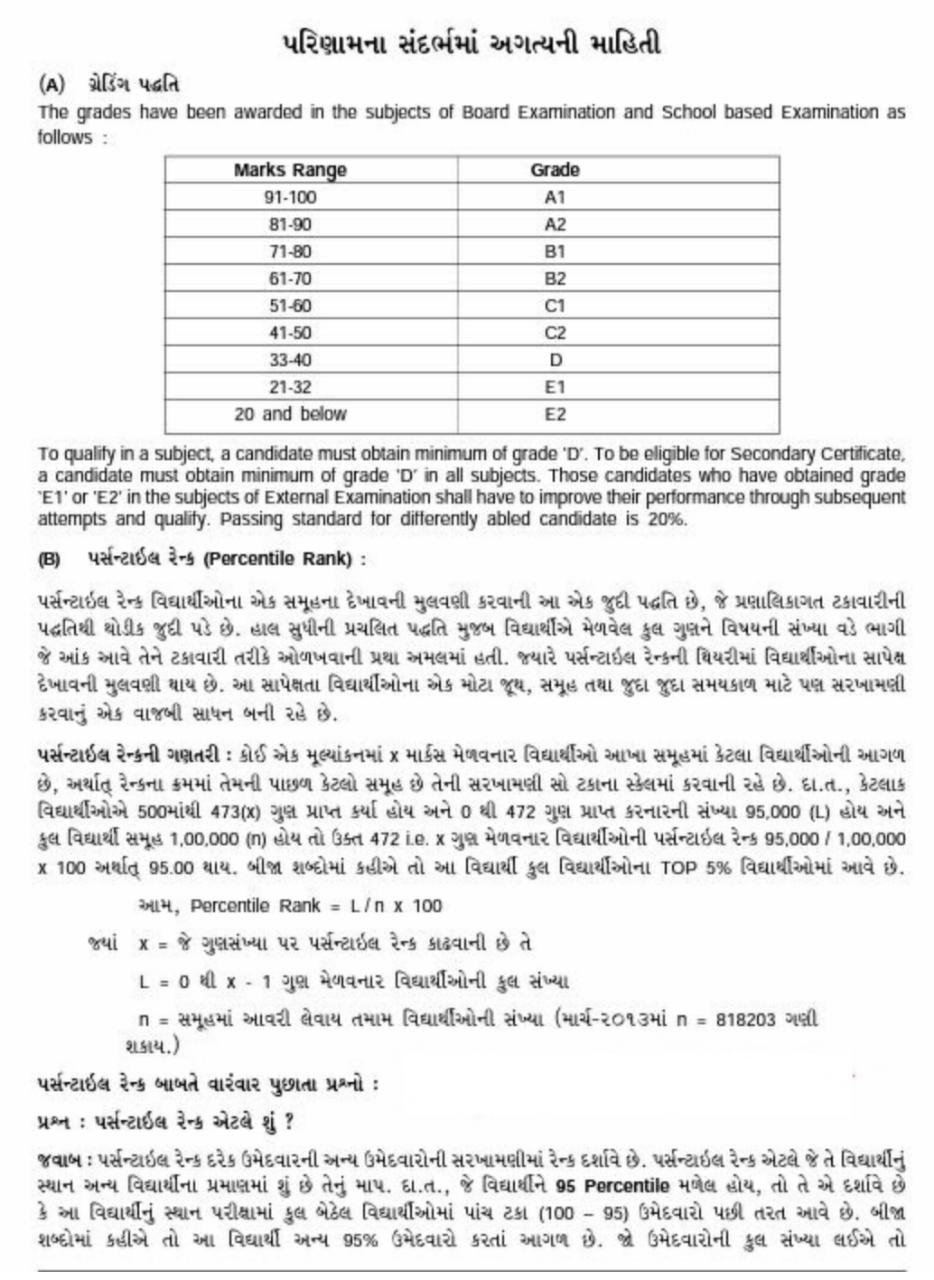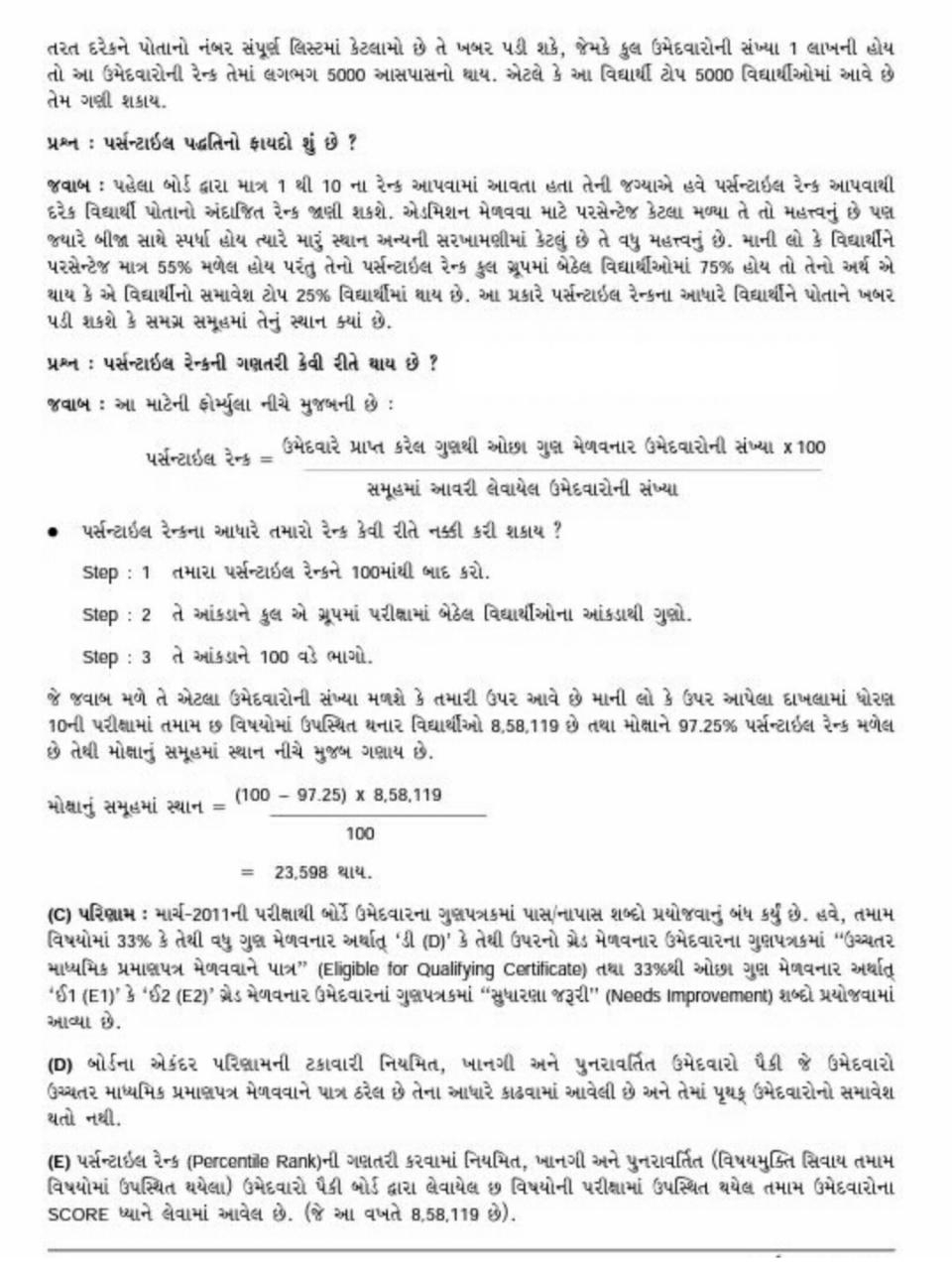# How to Calculate Percentile Rank & Percentage

## How to Calculate Percentile Rank & Percentage

How to Calculate Percentile Rank & Percentage
“Percentile” is in everyday use, but there is no universal definition for it. The most common definition of a percentile is a number where a certain percentage of scores fall below that number. You might know that you scored 67 out of 90 on a test. But that figure has no real meaning unless you know what percentile you fall into. If you know that your score is in the 90th percentile, that means you scored better than 90% of people who took the test.

Percentiles are commonly used to report scores in tests, like the SAT, GRE and LSAT. for example, the 70th percentile on the 2013 GRE was 156. That means if you scored 156 on the exam, your score was better than 70 percent of test takers.

The 25th percentile is also called the first quartile.
The 50th percentile is generally the median (if you’re using the third definition—see below).
The 75th percentile is also called the third quartile.
The difference between the third and first quartiles is the interquartile range.

2. Percentile Rank

The word “percentile” is used informally in the above definition. In common use, the percentile usually indicates that a certain percentage falls below that percentile. For example, if you score in the 25th percentile, then 25% of test takers are below your score. The “25” is called the percentile rank. In statistics, it can get a little more complicated as there are actually three definitions of “percentile.” Here are the first two (see below for definition 3), based on an arbitrary “25th percentile”:

Definition 1: The nth percentile is the lowest score that is greater than a certain percentage (“n”) of the scores. In this example, our n is 25, so we’re looking for the lowest score that is greater than 25%.

Definition 2: The nth percentile is the smallest score that is greater than or equal to a certain percentage of the scores. To rephrase this, it’s the percentage of data that falls at or below a certain observation. This is the definition used in AP statistics. In this example, the 25th percentile is the score that’s greater or equal to 25% of the scores.

They may seem very similar, but they can lead to big differences in results, although they are both the 25th percentile rank. Take the following list of test scores, ordered by rank:3. How to Find a Percentile

Sample question: Find out where the 25th percentile is in the above list.

Step 1: Calculate what rank is at the 25th percentile. Use the following formula:
Rank = Percentile / 100 * (number of items + 1)
Rank = 25 / 100 * (8 + 1) = 0.25 * 9 = 2.25.
A rank of 2.25 is at the 25th percentile. However, there isn’t a rank of 2.25 (ever heard of a high school rank of 2.25? I haven’t!), so you must either round up, or round down. As 2.25 is closer to 2 than 3, I’m going to round down to a rank of 2.

Step 2: Choose either definition 1 or 2:
Definition 1: The lowest score that is greater than 25% of the scores. That equals a score of 43 on this list (a rank of 3).
Definition 2: The smallest score that is greater than or equal to 25% of the scores. That equals a score of 33 on this list (a rank of 2).

Depending on which definition you use, the 25th percentile could be reported at 33 or 43! A third definition attempts to correct this possible misinterpretation:

Definition 3: A weighted mean of the percentiles from the first two definitions.

In the above example, here’s how the percentile would be worked out using the weighted mean:

Multiply the difference between the scores by 0.25 (the fraction of the rank we calculated above). The scores were 43 and 33, giving us a difference of 10:
(0.25)(43 – 33) = 2.5
Add the result to the lower score. 2.5 + 33 = 35.5
In this case, the 25th percentile score is 35.5, which makes more sense as it’s in the middle of 43 and 33.

In most cases, the percentile is usually definition #1. However, it would be wise to double check that any statistics about percentiles are created using that first definiti..